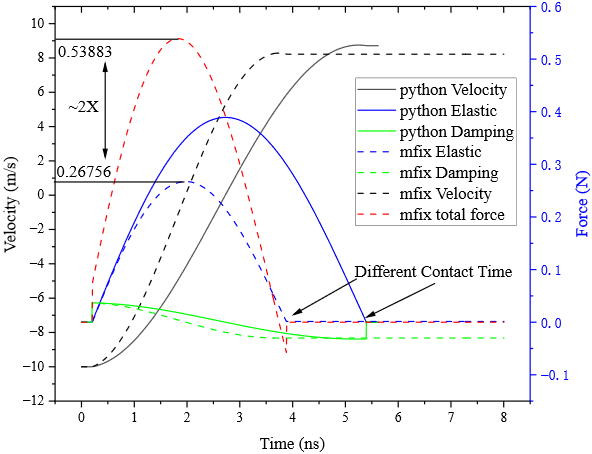# Issues with LSD Model in Particle Contact Simulation, how to avoid simultaneous contact between a single particle and multiple facets on a plane

Hello everyone,

I am currently working on simulating particle contact within particle layers using the LSD model. Initially, I’m trying to confirm these parameters through a single particle-wall contact, where I’ve used parameters obtained from my experimental data. My goal is to validate the DEM parameters for the next simulation.

I’ve attached my simulation program in the compressed package provided:
origin_2023-09-20T183307.442718.zip (2.2 MB)

In theory, according to the LSD model, when we don’t consider gas effects, the post-collision velocity should be equal to the incident velocity multiplied by the recovery coefficient. However, I’ve noticed that the post-collision speed is consistently lower than expected.

To investigate this issue, I’ve been monitoring the contact force in each “des” (discrete element simulation) loop, Save results in BACKGROUND_IC.csv, the code in cal_collision_wall_mod.f line 505 and line 533:
des_usr_var(1,LL) = OVERLAP_N
des_usr_var(2,LL) = -KN_DES_W * OVERLAP_N * NORMAL(2)
des_usr_var(3,LL) = -ETAN_DES_W * V_REL_TRANS_NORM * NORMAL(2)
des_usr_var(4,LL) = DIST(2)*FORCE_COH/SQRT(DISTSQ)
des_usr_var(5,LL) = FC(LL,2)

Initially, the contact force matches the theoretical calculations, as shown in the code excerpt:
FNORM ( : ) = -(KN_DES_W * OVERLAP_N * NORMAL( : ) + ETAN_DES_W * V_REL_TRANS_NORM * NORMAL( : ))——(in cal_collision_wall_mod.f line 462)

However, the total force becomes double what it should be in the following line:
FC(LL,: ) = FC(LL,: ) + FNORM( : ) + FTAN( : )—— (in cal_collision_wall_mod.f line 503)

As a result, the post-collision velocity is less than what is expected based on the recovery coefficient, and the total contact time is shorter than the theoretical contact time predicted by the LSD model. Visual representations of these force and velocity changes are provided in the attached figure. I used Python to calculate a similar process here, where the time step and force calculation formulas are the same as those in MFIX:I’ve identified that this issue arises because each particle with a “cell_id” contacts three facets in the simulation, causing the force to be redundantly calculated. The code is on the following line：
DO CELL_COUNT = 1, facets_at_dg(cell_id)%count——（in cal_collision_wall_mod.f line 187）

I have a few questions:

1. Why is this problem occurring, and how can it be resolved?
2. What is the significance of “cell_id” and “facets_at_dg” in this context?

I would appreciate any insights or suggestions for modifying my approach. If you need more information or have further questions, please feel free to ask. Thank you for your assistance!

Can you try to run again without the bottom wall BC. MFiX has some default BC wall that I think is the source of the issue. If you have a wall BC defined at the MFiX box boundary, we end up with a “double wall” in the sense that both the default wall and the defined wall BC are triangulated so there are 2 STL triangles colliding with the particle. We should either disable the default wall or provide an option to opt out.

Thank you for your suggestion. I tried to remove the bottom wall boundary, and now the results look very reasonable, just like the figure below. Thank you again.